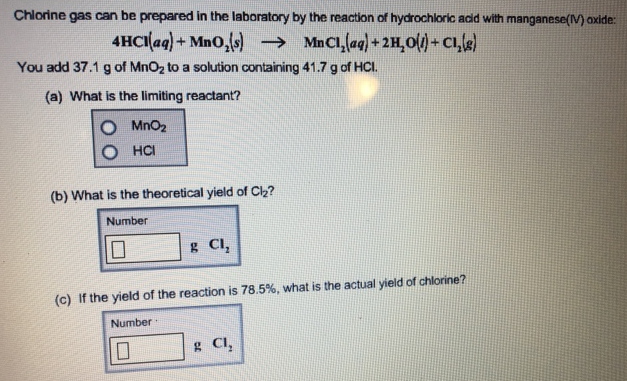# Problem: Chlorine gas can be prepared in the laboratory by the reaction of hydrochloric add with manganese(IV) oxide: 4HCI (aq) + MnO2 (s) → MnCI2 (aq) + 2H2O (l) + CI2 (g) You add 37.1 g of MnO2 to a solution containing 41.7 g of HCI. (a) What is the limiting reactant?                   • MnO2                 • HCl(b) What is the theoretical yield of CI2? (c) If the yield of the reaction is 78.5%, what is the actual yield of chlorine?

###### FREE Expert Solution
89% (37 ratings)###### Problem Details

Chlorine gas can be prepared in the laboratory by the reaction of hydrochloric add with manganese(IV) oxide:

4HCI (aq) + MnO2 (s) → MnCI2 (aq) + 2H2O (l) + CI2 (g)

You add 37.1 g of MnO2 to a solution containing 41.7 g of HCI.

(a) What is the limiting reactant?

• MnO2

• HCl

(b) What is the theoretical yield of CI2

(c) If the yield of the reaction is 78.5%, what is the actual yield of chlorine?